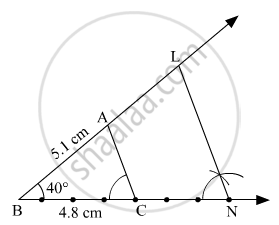# ∆Abc ~ ∆Lbn. in ∆Abc, Ab = 5.1cm, ∠B = 40°, Bc = 4.8 Cm, a C L N = 4 7 . Construct ∆Abc and ∆Lbn. - Geometry

Sum

∆ABC ~ ∆LBN. In ∆ABC, AB = 5.1cm, ∠B = 40°, BC = 4.8 cm,$\frac{AC}{LN} = \frac{4}{7}$. Construct ∆ABC and ∆LBN.

#### Solution

Steps of Construction:
Step 1: Draw ∆ABC such that AB = 5.1 cm, ∠B = 40°, BC = 4.8 cm.
Step 2: Divide segment BC into 4 equal parts.
Step 3: Mark point N on ray BC such that length of BN is seven times of each part of segment BC.
Step 4: Draw a line parallel to side AC, through N. Name the point where the parallel line intersects ray BA as L.Here, ∆ABC ~ ∆LBN.

Concept: Division of a Line Segment
Is there an error in this question or solution?